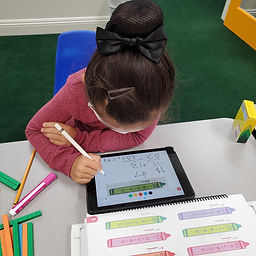## Ms. Raejean

### Target 1​

###### Lesson Type:

Continuation

Algebra

:

Variable

Determine the unknown number in an equation relating three whole numbers.

###### 1:

Use understandings of arithmetic to solve problems (where one part of the equation is given and the answer).

###### 2:

Understand the inverse relationship between addition and subtraction.

3rd

###### Vocabulary:

Multi-step, Equation, Equal, Unknown Numbers, Variable, Inverse Operation

Activities:

Students continued to solve multi-step equations. Problems were solved left to right. Then, students solved for the unknown number using inverse operations.

Next, students balanced equations. The equal sign indicates that both the left and right side are equal.

Ex. 48 - 12 = 46 - __

Step 1: 48 - 12 = 36

Step 2: 36 = 46 - __

Step 3: 46 - 36 = __

The unknown number is 10### Home Exploration

###### Guiding Questions:## Absent Students:

### Target 2

:

###### Vocabulary:

Activities:### Home Exploration

###### Guiding Questions:### Target 3

:

###### Vocabulary:

Activities:### Home Exploration